§3  A straight line on a plane

1. Equations and graphs of a straight line in a plane

 Equations and Graphics Description [ oblique cut ]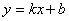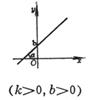k is the slope . If the intersection angle between the line and the x -axis is a , then 0 ￡ a < p .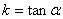b is the vertical intercept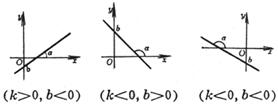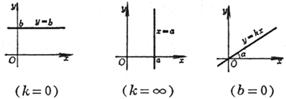[ intercept ]a , b are the intercepts on the x - axis and y -axis, respectively .   The line passes through the points A ( a , 0) and B (0, b ) .    Intersection angle with the x -axis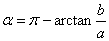or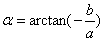[ dot slant ]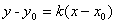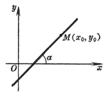k is the slope         The line passes through the point M ( x 0 , y 0 ) and intersects the x -axis at an angle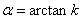equation with graphics Description [ two-point type ]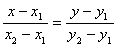or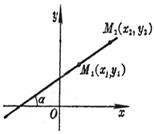The straight line passes through two points M 1 ( x 1 , y 1 ) and M 2 ( x 2,  y 2 ) ,    Intersection angle with the x -axis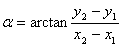[ General formula ]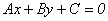( A , B , C are constants, A and B are not zero at the same time )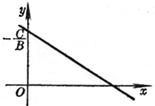slope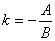Longitudinal intercept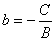[ Note ] The general equation can be transformed into the above four forms as needed [ parameter ]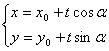or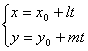( - ∞ < t < ∞ )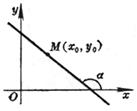slope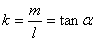The straight line passes through the point M ( x 0 , y 0 ) and the intersection angle with the x -axis is a [ Polar Coordinate Form ]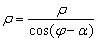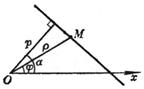O is the pole, Ox is the polar axis, p is the distance from the pole to the line . a is the angle between the polar axis and the vertical line leading from the pole to the line ( positive counterclockwise ) , j is any point on the line Polar angle of M, r is the vector radius of point M [ normal type ]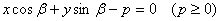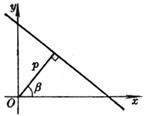p is the length of the normal line ( the length of the vertical line from the origin O to the line ) , b is the intersection angle between the normal line and the x -axis, and p and b are called the position parameters of the line . [ Note ] The general formula of a straight line can be transformed into a normal formula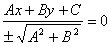In the formula, it is called the normalization factor of the straight line. When C < 0 ( or C = 0 and B > 0) , take the positive sign; when C > 0 ( or C = 0 and B < 0) , take the negative sign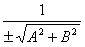[ vector ]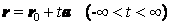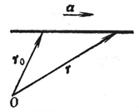The line passes through the end point of the vector radius r 0 and is parallel to the known vector a equation with graphics Description [ plural ]    ( a )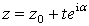( b )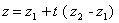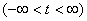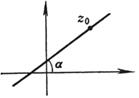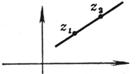(a) The line passes through the point z 0 and intersects the x -axis at an angle a             ( b ) The straight line passes through two points z 1 , z 2 ( t is a real parameter )

The relationship between points and lines on a plane

 Equations and Graphics Calculation formula and description [ distance of dotted line ] normal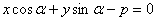general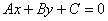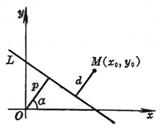d method =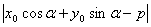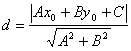where d is the distance from point M ( x 0 , y 0 ) to straight line L [ Included angle between two straight lines ] L 1     A 1 x + B 1 y + C 1 = 0      The slope is k 1 L 2     A 2 x + B 2 y + C 2 = 0      The slope is k 2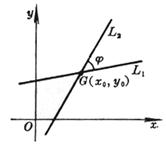is the angle between the two straight lines ( positive when counterclockwise from L 1 to L 2 ), and is the intersection of the two straight lines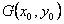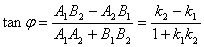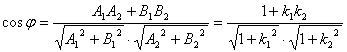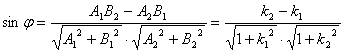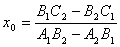;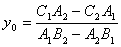In particular, when ( or ) , L 1 // L 2 ;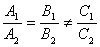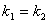At that time , L 1 coincides with L 2 ;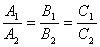When A 1 A 2 + B 1 B 2 = 0 ( or 1 + k 1 k 2 = 0) , L 1 ⊥ L 2 Equations and Graphics Calculation formula and description [ Conditions for straight line bundle × three straight lines co-point ]        L l    ( A 1 x + B 1 y + C 1 ) + l ( A 2 x + B 2 y + C 2 ) = 0, ( l is a parameter, - ￥ < l < ￥ )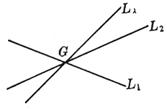For a certain value of l, L l represents a straight line passing through the intersection point G of two straight lines ( L 1 and L 2 ) . When l takes all values, the whole of the straight lines that L l represents passing through G is called a straight line bundle, G is called the vertex ( or center ) of the bundle of lines.        Let L 3 be A 3 x + B 3 y + C 3 = 0 , then the condition for the three straight lines L 1 , L 2 , and L 3 to have the same point is the determinantIf the equation of the two straight lines is given in the normal form, then | l | is the ratio of the distance between any point on the straight line L l and the two given straight lines, and the lines corresponding to l = 1 and l = - 1 are the given two straight lines Bisector of included angle